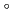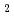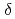The solubility of oxygen in liquid sodium was determined by vacuum distillation. Oxygen concentration was controlled by a cold trap in a dynamic sodium loop, and sodium was sampled from an expansion tank by dipping cup method and analysed by on line vacuum distillation method. A least-squares fit of the 37 experimental data over the range from 150 to 250C of cold trap temperature yielded a correlating equation of log S = 6.118-(2383/T) where S= wt ppm of oxygen in sodium assumed to be NaO. T = absolute temperature (K) The heat of solutionH of NaO in liquid sodium was calculated from this equation to be 10902 cal/mole. The reproducibility of results was obtained at equilibrium temperature of 150, 200, and 250C with a standard deviation of 1.2, 1.8, and 4.1 ppm, respectively. The oxygen analysis results with cold trapping sodium from various sodium test loops in Oarai Engineering Center almost correspond with the solubility curve of this work.